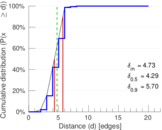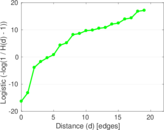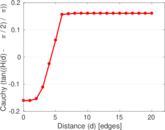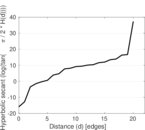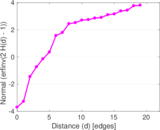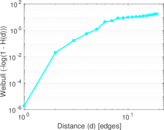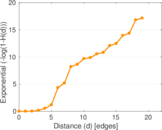# LiveJournal

This is the bipartite network of LiveJournal users and their group memberships.

 Code `LG` Internal name `livejournal-groupmemberships` Name LiveJournal Data source http://socialnetworks.mpi-sws.org/data-imc2007.html AvailabilityDataset is available for download Consistency checkDataset passed all tests Category Affiliation network Node meaning User, group Edge meaning Membership Network formatBipartite, undirected Edge typeUnweighted, no multiple edges

## Statistics

 Size n = 10,690,276 Left size n1 = 3,201,203 Right size n2 = 7,489,073 Volume m = 112,307,385 Wedge count s = 2,703,813,727,685 Claw count z = 383,401,902,790,995,904 Cross count x = 7.228 97 × 1022 Maximum degree dmax = 1,053,676 Maximum left degree d1max = 300 Maximum right degree d2max = 1,053,676 Average degree d = 21.011 1 Average left degree d1 = 35.082 9 Average right degree d2 = 14.996 2 Fill p = 4.684 54 × 10−6 Size of LCC N = 10,501,721 Diameter δ = 28 50-Percentile effective diameter δ0.5 = 4.289 86 90-Percentile effective diameter δ0.9 = 5.703 44 Median distance δM = 5 Mean distance δm = 4.732 86 Gini coefficient G = 0.852 761 Balanced inequality ratio P = 0.150 057 Left balanced inequality ratio P1 = 0.273 067 Right balanced inequality ratio P2 = 0.084 634 3 Relative edge distribution entropy Her = 0.821 064 Power law exponent γ = 1.858 35 Degree assortativity ρ = −0.149 431 Degree assortativity p-value pρ = 0.000 00 Spectral norm α = 1,912.07

## Plots

### Degree distribution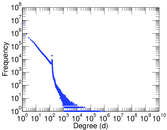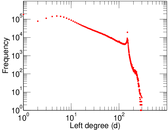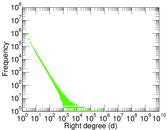### Cumulative degree distribution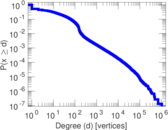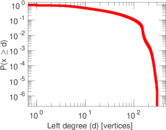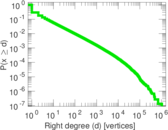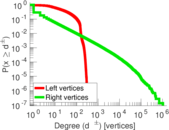### Lorenz curve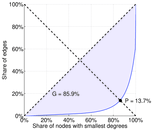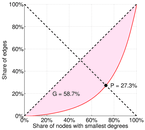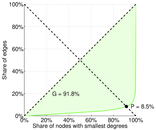### Spectral distribution of the adjacency matrix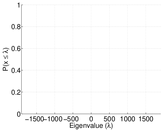### Spectral distribution of the normalized adjacency matrix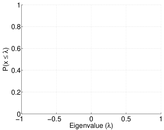### Spectral distribution of the Laplacian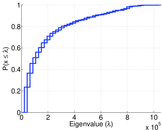### Spectral graph drawing based on the adjacency matrix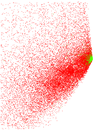### Spectral graph drawing based on the normalized adjacency matrix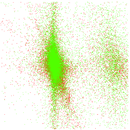### Degree assortativity### Hop distribution